Courses

# Linear Programming (Part - 1) Electronics and Communication Engineering (ECE) Notes | EduRev

## Electronics and Communication Engineering (ECE) : Linear Programming (Part - 1) Electronics and Communication Engineering (ECE) Notes | EduRev

The document Linear Programming (Part - 1) Electronics and Communication Engineering (ECE) Notes | EduRev is a part of Electronics and Communication Engineering (ECE) category.
All you need of Electronics and Communication Engineering (ECE) at this link: Electronics and Communication Engineering (ECE)

LINEAR PROGRAMMING

It can be recalled from the Two Mines example that the conditions for a mathematical model to be a linear program (LP) were:

• all variables continuous (i.e. can take fractional values)
• a single objective (minimize or maximize)
• the objective and constraints are linear i.e. any term is either a constant or a constant multiplied by an unknown.

LP's are important - this is because:

• many practical problems can be formulated as LP's
• there exists an algorithm (called the simplex algorithm) which enables us to solve LP's numerically relatively easily

We will return later to the simplex algorithm for solving LP's but for the moment we will concentrate upon formulating LP's.

Some of the major application areas to which LP can be applied are:

• Work scheduling
• Production planning & Production process
• Capital budgeting
• Financial planning
• Blending (e.g. Oil refinery management)
• Farm planning
• Distribution
• Multi-period decision problems
• Inventory model
• Financial models
• Work scheduling

Note that the key to formulating LP's is practice. However a useful hint is that common objectives for LP's are maximize profit/minimize cost.

There are four basic assumptions in LP:

• Proportionality
• The contribution to the objective function from each decision variable is proportional to the value of the decision variable (The contribution to the objective function from making four soldiers (4x\$3=\$12) is exactly four times the contribution to the objective function from making one soldier (\$3))
• The contribution of each decision variable to the LHS of each constraint is proportional to the value of the decision variable (It takes exactly three times as many finishing hours (2hrsx3=6hrs) to manufacture three soldiers as it takes to manufacture one soldier (2 hrs))
• The contribution to the objective function for any decision variable is independent of the values of the other decision variables (No matter what the value of train (x2), the manufacture of soldier (x1) will always contribute 3x1 dollars to the objective function)
• The contribution of a decision variable to LHS of each constraint is independent of the values of other decision variables (No matter what the value of x1, the manufacture of x2 uses x2 finishing hours and x2 carpentry hours)

1st implication: The value of objective function is the sum of the contributions from each decision variables.
2nd implication: LHS of each constraint is the sum of the contributions from each decision variables.

• Divisibility
• Each decision variable is allowed to assume fractional values. If we actually can not produce a fractional number of decision variables, we use IP (It is acceptable to produce 1.69 trains)
• Certainty
• Each parameter is known with certainty

FORMULATING LP

Giapetto Example

(Winston 3.1, p. 49)

Giapetto's wooden soldiers and trains. Each soldier sells for \$27, uses \$10 of raw materials and takes \$14 of labor & overhead costs. Each train sells for \$21, uses \$9 of raw materials, and takes \$10 of overhead costs. Each soldier needs 2 hours finishing and 1 hour carpentry; each train needs 1 hour finishing and 1 hour carpentry. Raw materials are unlimited, but only 100 hours of finishing and 80 hours of carpentry are available each week. Demand for trains is unlimited; but at most 40 soldiers can be sold each week. How many of each toy should be made each week to maximize profits?

Decision variables completely describe the decisions to be made (in this case, by Giapetto). Giapetto must decide how many soldiers and trains should be manufactured each week. With this in mind, we define:
x1 = the number of soldiers produced per week x2 = the number of trains produced per week

Objective function is the function of the decision variables that the decision maker wants to maximize (revenue or profit) or minimize (costs). Giapetto can concentrate on maximizing the total weekly profit (z).
Here profit equals to (weekly revenues) – (raw material purchase cost) – (other variable costs). Hence Giapetto’s objective function is:

Maximize z = 3x1 + 2x2

Constraints show the restrictions on the values of the decision variables. Without constraints Giapetto could make a large profit by choosing decision variables to be very large.

Here there are three constraints:

Finishing time per week

Carpentry time per week

Weekly demand for soldiers

Sign restrictions are added if the decision variables can only assume nonnegative values (Giapetto can not manufacture negative number of soldiers or trains!)

All these characteristics explored above give the following Linear Programming (LP) model
max z = 3x1 + 2x2
(The Objective function)
s.t. 2x1 + x2 ≤ 100
(Finishing constraint)
x+x2 ≤ 80
(Carpentry constraint)
x1≤ 40
(Constraint on demand for soldiers)
x1, x2 > 0
(Sign restrictions)
A value of (x1, x2) is in the feasible region if it satisfies all the constraints and sign restrictions.
Graphically and computationally we see the solution is (x1, x2) = (20, 60) at which z =
180. (Optimal solution)

Report

The maximum profit is \$180 by making 20 soldiers and 60 trains each week. Profit is limited by the carpentry and finishing labor available. Profit could be increased by buying more labor.

(Winston 3.2, p.61)
Dorian makes luxury cars and jeeps for high-income men and women. It wishes to advertise with 1 minute spots in comedy shows and football games. Each comedy spot costs \$50K and is seen by 7M high-income women and 2M high-income men. Each football spot costs \$100K and is seen by 2M high-income women and 12M high-income men. How can Dorian reach 28M high-income women and 24M high-income men at the least cost?

The decision variables are
x1 = the number of comedy spots
x2 = the number of football spots
The model of the problem:
min z = 50x1 + 100x2
st 7x1 + 2x2 ≥ 28
2x+ 12x2
24
x1, x2 ≥ 0

The graphical solution is z = 320 when (x1, x2) = (3.6, 1.4). From the graph, in this problem rounding up to (x1, x2) = (4, 2) gives the best integer solution.

Report

The minimum cost of reaching the target audience is \$400K, with 4 comedy spots and 2 football slots. The model is dubious as it does not allow for saturation after repeated viewings.

Diet Example

(Winston 3.4., p. 70)
Ms. Fidan’s diet requires that all the food she eats come from one of the four “basic food groups“. At present, the following four foods are available for consumption: brownies, chocolate ice cream, cola, and pineapple cheesecake. Each brownie costs 0.5\$, each scoop of chocolate ice cream costs 0.2\$, each bottle of cola costs 0.3\$, and each pineapple cheesecake costs 0.8\$. Each day, she must ingest at least 500 calories, 6 oz of chocolate, 10 oz of sugar, and 8 oz of fat. The nutritional content per unit of each food is shown in Table. Formulate an LP model that can be used to satisfy her daily nutritional requirements at minimum cost.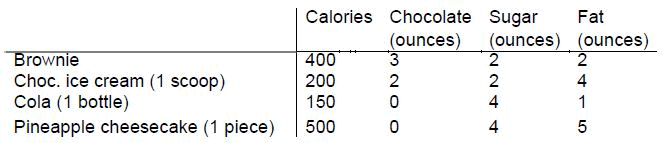The decision variables:
x1: number of brownies eaten daily
x2: number of scoops of chocolate ice cream eaten daily
x3: bottles of cola drunk daily
x4: pieces of pineapple cheesecake eaten daily
The objective function (the total cost of the diet in cents):
min w = 50x1 + 20x2 + 30x3 + 80x4

Constraints: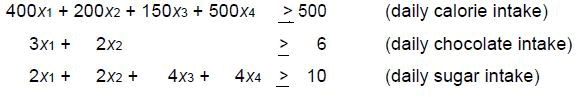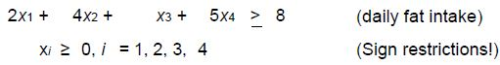Report

The minimum cost diet incurs a daily cost of 90 cents by eating 3 scoops of chocolate and drinking 1 bottle of cola (w = 90, x2 = 3, x3 = 1)

Post Office Example

(Winston 3.5, p.74)
A PO requires different numbers of employees on different days of the week. Union rules state each employee must work 5 consecutive days and then receive two days off. Find the minimum number of employees needed.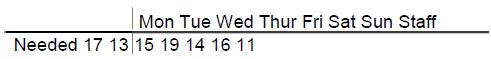The decision variables are xi (# of employees starting on day i)

Mathematically we must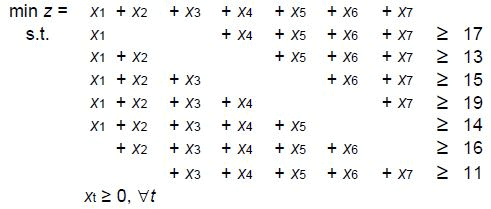The solution is (xi) = (4/3, 10/3, 2, 22/3, 0, 10/3, 5) giving z = 67/3.
We could round this up to (xi) = (2, 4, 2, 8, 0, 4, 5) giving z = 25 (may be wrong!). However restricting the decision var.s to be integers and using Lindo again gives (xi) = (4, 4, 2, 6, 0, 4, 3) giving z = 23.

Sailco Example

(Winston 3.10, p. 99)
Sailco must determine how many sailboats to produce in the next 4 quarters. The demand is known to be 40, 60, 75, and 25 boats. Sailco must meet its demands. At the beginning of the 1st quarter Sailco starts with 10 boats in inventory. Sailco can produce up to 40 boats with regular time labor at \$400 per boat, or additional boats at \$450 with overtime labor. Boats made in a quarter can be used to meet that quarter's demand or held in inventory for the next quarter at an extra cost of \$20.00 per boat.

The decision variables are for t = 1,2,3,4
xt = # of boats in quarter t built in regular time yt = # of boats in quarter t built in overtime
For convenience, introduce variables:
it = # of boats in inventory at the end quarter t dt = demand in quarter t
We are given that d1 = 40, d2 = 60, d3 = 75, d4 = 25, i0 = 10
xt ≤ 40,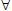t
By logic i= it-1+ x+ yt - dt,t.
Demand is met iff it ≥ 0,t
(Sign restrictions xt, yt ≥ 0,t)

We need to minimize total cost z subject to these three sets of conditions where z = 400 (x1 + x2 + x3 + x4) + 450 (y1 + y2 + y3 + y4) + 20 (i1 + i2 + i3 + i4)

Report:

Lindo reveals the solution to be (x1, x2, x3, x4) = (40, 40, 40, 25) and (y1, y2, y3, y4) = (0, 10, 35, 0) and the minimum cost of \$78450.00 is achieved by the schedule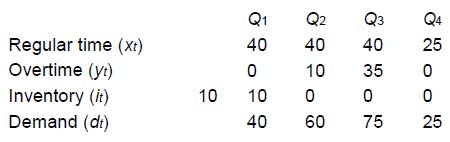Customer Service Level Example

(Winston 3.12, p. 108)
CSL services computers. Its demand (hours) for the time of skilled technicians in the next 5 months isIt starts with 50 skilled technicians at the beginning of January. Each technician can work 160 hrs/month. To train a new technician they must be supervised for 50 hrs by an experienced technician for a period of one month time. Each experienced technician is paid \$2K/mth and a trainee is paid \$1K/mth. Each month 5% of the skilled technicians leave. CSL needs to meet demand and minimize costs.

The decision variable is
xt = # to be trained in month t
We must minimize the total cost. For convenience let yt = # experienced tech. at start of tth month d= demand during month t
Then we must
min z = 2000 (y1+...+ y5) + 1000 (x+...+ x5)
subject to
160y- 50xt ≥ dt for t = 1,..., 5
y1 = 50, d1 = 6000, d2 = 7000, d3 = 8000, d4 = 9500, d5 = 11000
yt = .95yt-1 + xt-1 for t = 2,3,4,5
xt, yt ≥0

Offer running on EduRev: Apply code STAYHOME200 to get INR 200 off on our premium plan EduRev Infinity!

,

,

,

,

,

,

,

,

,

,

,

,

,

,

,

,

,

,

,

,

,

;